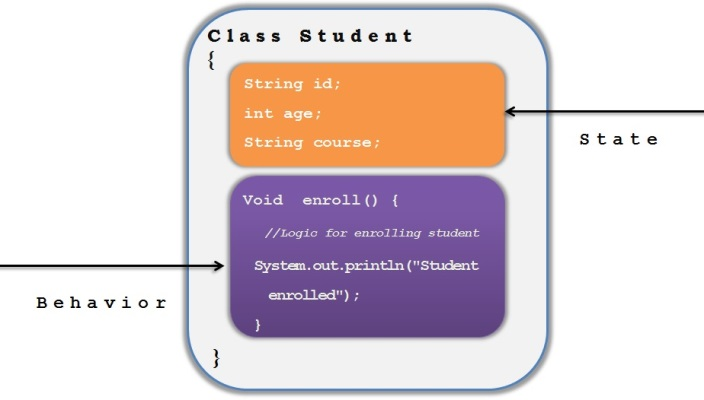# Class and objects in Java

### Let’s understand Class and objects in Java

##### Class

`Class `represents a real world entity which has both state and behavior which is applicable to all the objects of this Class.So `Class `is called as a blue print or a template using which each object will be created.

###### Example:

Create Student.java

1. Class Student{
2. String id;
3. int age;
4. String course;
5. Void  enroll(){
6.       //Logic for enrolling student
7.    System.out.println("Student enrolled");
8.  }
9. }
```Class Student{
String id;
int age;
String course;
Void  enroll(){
//Logic for enrolling student
System.out.println("Student enrolled");
}
}```

In the above class, we have a real world entity called “Student” which is represented by a class with its `states `like id,age and course and also `behavior `like enroll.A `class `will have local variables, instance variables and static variables and we need to have very clear understanding of these variables to use them as per the requirement.

###### Check Variables in Java article to understand the same.

A `class `can have any number of methods depending on our requirement.

In the above example, we have added only one method called “ enroll() “.

### Object

`Object `is an instance of class and generally objects are created using the `constructor`.

Each object holds the state and behavior specific to it

We can create any number of objects using a `class `and hence class is sometime referred as collection of objects.

Let’s create an Object of Student class as below

1. Student s1 = new Student();
`Student s1 = new Student();`

Now `object `is created and is referred by the reference variable called “s1”.

Once the `object `is created, we can use its reference variable to access the attributes and methods based on the access level defined.

```Check Heap and Stack article to understand where these objects gets created in Java
Check access modifiers  article to understand various access levels used in the class
```

We can set the state of this object as below

1. s1.id=123;
2. s1.age=18;
3. s1.course=”computers”;
```s1.id=123;
s1.age=18;
s1.course=”computers”;```

We can access the method to enroll this “s1” student as below

1. s1.enroll();
`s1.enroll();`

###### Complete example is as below

Student.java

1. Class Student{
2. String id;
3. int age;
4. String course;
5. Void  enroll(){
6. //Logic for enrolling student
7. System.out.println("Student enrolled");
8.  }
9. }
```Class Student{
String id;
int age;
String course;
Void  enroll(){
//Logic for enrolling student
System.out.println("Student enrolled");
}
}```

StudentManager.java

1. Class StudentManager{
2.
3. public static void main(String []args) {
4. Student s1 = new Student();
5. s1.id=123;
6. s1.age=18;
7. s1.course=”computers”;
8. s1.enroll();
9. }
10. }
```Class StudentManager{

public static void main(String []args) {
Student s1 = new Student();
s1.id=123;
s1.age=18;
s1.course=”computers”;
s1.enroll();
}
}```